# Introduction to the hpiR package

### Introduction

This vignette provides an introduction to using the hpiR package for estimating house price indexes. At the current time, this package (version 0.2.0) offers two approaches to estimate house price indexes – repeat transactions and hedonic price models. Future versions will incorporate additional methodological options. More information on this package’s development can be found at www.github.com/andykrause/hpiR

A detailed explanation of the class and method structure employed in this package can be found in the Classes in hpiR vignette in this package, or at: https://github.com/andykrause/hpiR/blob/master/vignettes/classstructure.Rmd

#### Terminology

A bit of terminology:

• Index: a time-series of values estimating tracking the movement of prices over time with a base period value of 100
• Series: progression of increasing longer indexes
• Model: statistical or other type of mathematical equation or algorithm used to generate the raw coefficient (or other) estimates that are converted into the scaled (base = 100) index values
• Accuracy: ability of an index to represent the actual market movements
• Volatility: measure of the period to period movement in index values
• Smooth: reduce volatility with a rolling average or similar smoothing process
• Revision: amount that the index value for a specific period moves over the course of a series

In general, this vignette takes the following path through the functionality provided by hpiR as this is imagined to most closely approximate a real-world application of the package to real estate market data.

1. Data preparation
2. Modeling
3. Index creation
4. Analysis: Index-level
5. Series creation
6. Analysis: Series-level

### Load Package

Begin by loading the hpiR packages (assuming that you’ve already downloaded it from: www.github.com/andykrause/hpiR or from CRAN (forthcoming).

  library(hpiR)

### Data

This package provides two sample datasets to work with. The first, seattle_sales, includes over 43,000 sales of single family homes and townhouses within the City of Seattle over the 2010 to 2016 time frame. The second, ex_sales is a subset of the first, encompassing only the central area of the city. The source of the data is the King County Assessor’s office.1 The data is open and free to share.

The data can be loaded with the data(seattle_sales) and data(ex_sales) commands. We will work with the ex_sales object in this vignette.

  data(seattle_sales)

data(ex_sales)

All potential models, indexes and series that can be created with hpiR require the transaction date to be converted to a numeric and relative time period. Relative, here, means relative to the base period which is assumed to be the first period in the transaction data.

The dateToPeriod() function will convert R date fields into relative time periods based on a periodicity of choice. The available periodicity options are:

• “yearly”
• “quarterly”
• “monthly”
• “weekly”

with “yearly” as the default. In addition to the transaction data (trans_df) and the period type selection (periodicity), the dateToPeriod() function also requires an input telling it which field in the data contains the transaction date (date).

  sales_hdf <- dateToPeriod(trans_df = ex_sales,
date = 'sale_date',
periodicity = 'monthly')

The default specification of dateToPeriod() will use the minimum and maximum dates in the data provided to it as the lower and upper time boundaries for the modeling and index creation that follow. You can, however, set custom dates using the min_date and max_date arguments. For example, let’s assume that we want to estimate an index that covers the entire transaction period plus one month on either side (in this case from December 2009 to January 2017). We add these dates as alternate minimums and maximums. You see a warning telling you that there are empty periods – periods in which there are no transactions.

  sales_hdf <- dateToPeriod(trans_df = ex_sales,
date = 'sale_date',
periodicity = 'monthly',
min_date = as.Date('2009-12-01'),
max_date = as.Date('2016-12-31'))
## Your choice of periodicity resulted in 1 empty periods out of 85 total periods.

If the dates that are provided as alternative minimums and maximums are within the range of transaction dates in the data, there are two options to what can happen. The first, and default, is that the minimums and maximums will be adjusted to be the actual minimum and maximum dates in the transactions. A warning will let you know that this has happened.

  sales_hdf <- dateToPeriod(trans_df = ex_sales,
date = 'sale_date',
periodicity = 'monthly',
min_date = as.Date('2010-12-01'),
max_date = as.Date('2015-12-31'))
## Supplied "min_date" is greater than minimum of transactions. Adjusting.
## Supplied "max_date" is less than maximum of transactions. Adjusting.

Alternatively, you can use the min_date and max_date arguments to “clip”" your dataset to a particular time frame by setting adj_type = "clip". The default, adj_type = "move", simply moves it to the minimum or maximum in the data as shown above. The clipping functionality allows you to create an index for a subset of the time period covered by your data.

  sales_hdf_clip <- dateToPeriod(trans_df = ex_sales,
date = 'sale_date',
periodicity = 'monthly',
min_date = as.Date('2010-12-01'),
max_date = as.Date('2015-12-31'),
adj_type = 'clip')
## Supplied "min_date" date is greater than minimum of transactions. Clipping transactions.
## Supplied "max_date" is less than maximum of transactions. Clipping transactions.

For the sake of the remainder of this analysis, let’s allow the defaults to set the time boundaries based on the data itself (we will use the sales_hdf object going forward).

We now have a dataset that includes two new, standardized fields (trans_date and trans_period) indicating the date of transaction and the period of each transaction relative to the first transaction in the data (at a monthly periodicity), respectively. Note that these standardized fields are required for the processes that follow.

Additional information on the full extent of the periods as well as their numeric and date values can be found in the "period_table" attribute of the sales_hdf object or accessed by:

  head(attr(sales_hdf, 'period_table'))
##       name  numeric period
## 1 2010-Jan 2010.000      1
## 2 2010-Feb 2010.083      2
## 3 2010-Mar 2010.167      3
## 4 2010-Apr 2010.250      4
## 5 2010-May 2010.333      5
## 6 2010-Jun 2010.417      6

Finally, information on the minimum date, maximum date and the periodicity can also be found in the attributes of hpidata objects.

### Repeat Transactions (Sales) Model

Let’s start by creating an index using a repeat-transactions model (often referred to as a repeat-sales or a sale-resale model), one of the most popular approaches to create a house price index.

#### Data Prep

First, we use the rtCreateTrans() function to convert the transaction data (the hpidata object) into a data.frame of repeat transactions (properties that have transacted multiple times in the 2010 to 2016 period.) The rtCreateTrans() function requires a unique property id field, a unique transaction id field and a field denoting the transaction price.

  sales_rtdf <- rtCreateTrans(trans_df = sales_hdf,
prop_id = 'pinx',
trans_id = 'sale_id',
price = 'sale_price')


This function creates a data.frame with eight fields: the property id, the time period for the first transaction and the second transaction, the prices for the two tranactions, the unique transaction ids for both and a unique id for the pair. The resulting object has a class of rtdata (inheriting from hpidata and data.frame).

prop_id period_1 period_2 price_1 price_2 trans_id1 trans_id2 pair_id
..0007600057 56 80 520000 625000 2014..23738 2016..28612 1
..0216000010 29 47 1063000 1300000 2012..12112 2013..36837 2
..0342000250 40 78 626800 751000 2013..11548 2016..17781 3
..0342000570 15 57 295000 429000 2011..4885 2014..27574 4
..0342000570 15 78 295000 607000 2011..4885 2016..18557 5
..0342000570 57 78 429000 607000 2014..27574 2016..18557 6

In the event of more than two transactions of the same property, all pairs of transactions are created by default – sales 1 to 2, 1 to 3 and 2 to 3. If you’d like to only use sequential transactions as pairs – sales 1 to 2 and 2 to 3, for example – you can set seq_only = TRUE (default is FALSE).

  sales_rtdf_so <- rtCreateTrans(trans_df = sales_hdf,
prop_id = 'pinx',
trans_id = 'sale_id',
price = 'sale_price',
seq_only = TRUE)
prop_id period_1 period_2 price_1 price_2 trans_id1 trans_id2 pair_id
..0007600057 56 80 520000 625000 2014..23738 2016..28612 1
..0216000010 29 47 1063000 1300000 2012..12112 2013..36837 2
..0342000250 40 78 626800 751000 2013..11548 2016..17781 3
..0342000570 15 57 295000 429000 2011..4885 2014..27574 4
..0342000570 57 78 429000 607000 2014..27574 2016..18557 5
..0345000485 8 31 622000 517000 2010..15338 2012..17939 6

#### Modeling

Next, we use the rtdata object to estimate a repeat transactions model. In this simple example we will use the “base” estimator (a simple OLS) with a logged dependent variable (log_dep = TRUE). Models may also be estimated via a robust regression model (estimator = "robust") or a weighted OLS model (estimator = "weighted") as originally suggested by Case and Shiller (1987).

The hpiModel() function is an S3 method that will dispatch on the class of the object that is passed to its hpi_df argumet. In this case that class is rtdata signifying that the data is in repeat-transaction format. Again, we’ll use a base estimator here with a logged dependent variable. The hpiModel() function returns an object of class hpimodel that includes the full regression model results, extracted and cleaned coefficients as well as additional information on the approach.

  rt_model <- hpiModel(hpi_df = sales_rtdf,
estimator = 'base',
log_dep = TRUE)

The objects created by regression models can be rather large, so by default the hpiModel() function trims some of the unnecessary results from the model estimation function(s). If you want full results you can set trim_model = FALSE.

  rt_full <- hpiModel(hpi_df = sales_rtdf,
estimator = 'base',
log_dep = TRUE,
trim_model = FALSE)

object.size(rt_model)
## 591816 bytes
object.size(rt_full)
## 1079744 bytes

#### Index Creation

We can then convert model results into an index with the modelToIndex() function. The output of this function is an objet of class hpiindex, which has a time-series object ($value slot, class ts) with an index value (base = 100) for each of the time periods estimated. Note that no smoothing is done at this time.  rt_index <- modelToIndex(model_obj = rt_model) If you want to limit the extent of the index (clip periods from the end of it) you can set the max_period argument within the modelToIndex() function to the last period you wish to have in the index.  rt_short <- modelToIndex(model_obj = rt_model, max_period = 50) length(rt_short$value)
##  50

A simple plot (with the plot.hpiindex() method) shows the results of this estimation.

  plot(rt_index)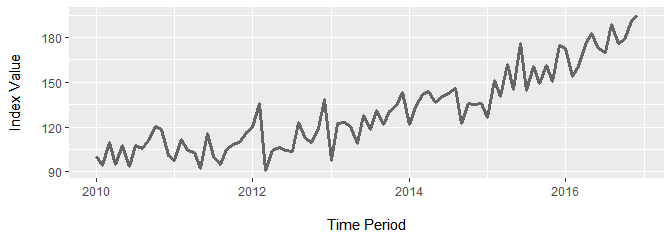In situations where there are empty periods in the modeling results (there was no or not enough data to estimate a value for a particular period), the modelToIndex() will impute (using the imputeTS package) the missing periods. For missing periods at the start of the index or before the first non-100 period, all periods are given a value of 100. For missing internal periods (those with estimated periods both before and after) a ‘stine’ interpolation is used and for those missing at the end a ‘last observation carried forward’ (LOCF) approach is used. The \$imputed slot in the hpiindex object will indicate which periods have been imputed.  rt_model_imp <- rt_full rt_model_imp$coefficients$coefficient[3:5] <- NA rt_index_imp <- modelToIndex(model_obj = rt_model_imp) ## Total of 3 period(s) imputed rt_index_imp$value[1:6]
##  100.00000  94.32403  93.77995  93.50346  93.37582  93.33960

rt_index_imp$imputed[1:6] ##  0 0 1 1 1 0 The plot function can show the imputed periods as well with show_imputed=TRUE.  rt_model_imp <- rt_full rt_model_imp$coefficients$coefficient[c(4, 7, 23, 55, 78, 83)] <- NA rt_index_imp <- modelToIndex(model_obj = rt_model_imp) ## Total of 6 period(s) imputed plot(rt_index_imp, show_imputed=TRUE)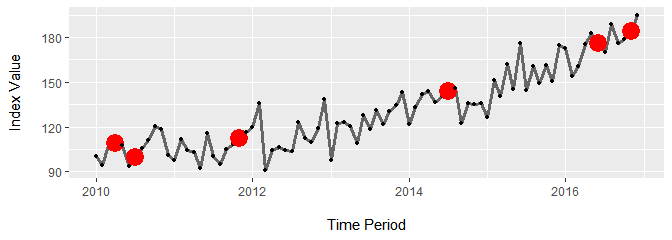#### Smoothing As we see from the plots of the indexes above, considerable volatility from one period to the next is common. Smoothing of indexes may be warranted as indexes resulting from raw model results often exhibit greater volatility than is actually occuring in the market. The smoothIndex() function will smooth via a moving average function, with small corrections to keep index length after smoothing. The amount of smoothing is controlled by the order argument – the number of periods over which to smooth. Note that the order is saved in the attributes of the indexsmooth object.  rt_smooth <- smoothIndex(index_obj = rt_index, order = 5) attr(rt_smooth, 'order') ##  5 The smoothIndex() function can be done ‘in_place’ such that the smoothed indexes are added to the larger hpiindex object.  rt_index <- smoothIndex(index_obj = rt_index, order = 7, in_place = TRUE) names(rt_index) ##  "name" "numeric" "period" "value" "imputed" "smooth" The in_place smoothing can be plotted by adding smooth=TRUE to the plot call.  plot(rt_index, smooth=TRUE)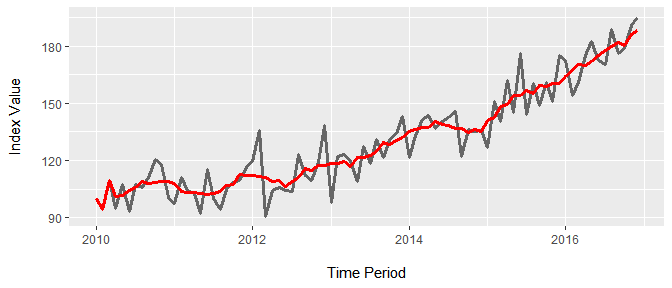#### Data to Index Wrapper A wrapper function, rtIndex(), will do all of the above taking it from a raw data.frame of transaction data through to the repeat transaction index creation and storing all results in a unified object of class hpi. There are three ‘levels of entry’ with the wrapper function. 1. You can give it a raw data.frame with your transactions and it will A) Add the periods; B) Calculate the repeat transactions; and C) Model the data and create the index. Under this approach trans_df is passed a raw data.frame along with the following arguments, some of which are optional (will revert to default if not given) and some of which are required (mostly field names): • periodicity (optional: default = ‘annual’) • min_date (optional: default = minimum of data) • max_date (optional: default = maximum of data) • adj_type (opt: default = ‘move’) • date (required) • price (required) • trans_id (required) • prop_id (required) • seq_only (optional: default = FALSE) • estimator (optional: default = ‘base’) • log_dep (optional: default = TRUE) • trim_model (optional: default = FALSE) • max_period (optional: default = maximum of index length) • smooth (optional: default = FALSE) • smooth_order (optional, default = 3)  rt_1 <- rtIndex(trans_df = ex_sales, periodicity = 'monthly', min_date = '2010-06-01', max_date = '2015-11-30', adj_type = 'clip', date = 'sale_date', price = 'sale_price', trans_id = 'sale_id', prop_id = 'pinx', seq_only = TRUE, estimator = 'robust', log_dep = TRUE, trim_model = TRUE, max_period = 48, smooth = FALSE) ## Supplied "min_date" date is greater than minimum of transactions. Clipping transactions. ## Supplied "max_date" is less than maximum of transactions. Clipping transactions. 1. You can provide a hpidata object (one that has been through the dateToPeriod() function). In this case, the following arguments are valid: • date (required) • price (required) • trans_id (required) • prop_id (required) • seq_only (optional: default = FALSE) • estimator (optional: default = ‘base’) • log_dep (optional: default = TRUE) • trim_model (optional: default = FALSE) • max_period (optional: default = maximum of index length) • smooth (optional: default = FALSE) • smooth_order (optional, default = 3)  sales_hdf <- dateToPeriod(trans_df = ex_sales, date = 'sale_date', periodicity = 'monthly')  rt_2 <- rtIndex(trans_df = sales_hdf, date = 'sale_date', price = 'sale_price', trans_id = 'sale_id', prop_id = 'pinx', seq_only = FALSE, estimator = 'weighted', log_dep = FALSE, trim_model = FALSE, max_period = 56, smooth = TRUE) 1. Finally, you can provide it an rtdata object (one that has been through the rtCreateTrans() function). Only the following arguments are meaningful in this case. • estimator (optional: default = ‘base’) • log_dep (optional: default = TRUE) • trim_model (optional: default = FALSE) • max_period (optional: default = maximum of index length) • smooth (optional: default = FALSE) • smooth_order (optional, default = 3)  sales_rtdf <- rtCreateTrans(trans_df = sales_hdf, prop_id = 'pinx', trans_id = 'sale_id', price = 'sale_price')  rt_3 <- rtIndex(trans_df = sales_rtdf, estimator = 'robust', log_dep = TRUE, trim_model = FALSE, max_period = 80, smooth = TRUE, smooth_order = 5) A plot method exists to cleanly plot hpi objects.  plot(rt_3, smooth=TRUE)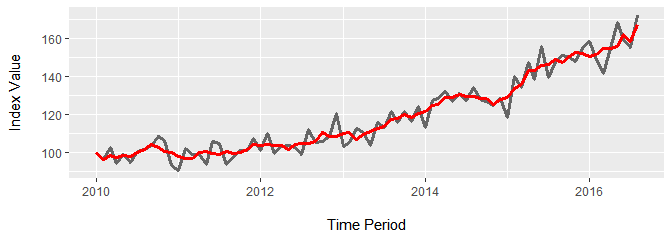### Hedonic Price Model Another option for developing a house price index is to use a hedonic price model. Similar to the repeat transaction model presented above, this process can be done stepwise or through a single wrapper function (hedIndex()). We’ll start with individual steps. First, we create a set of hedonic model ready data with the hedCreateTrans() function. Here we provide the raw transactions, along with field names for the property id, the transaction ids, the price and date. A periodicity (“yearly”, “quarterly”, “monthly” or “weekly”) is also required. Note that this function uses the dateToPeriod() discussed above and creates an object of class heddata inheriting from hpidata and data.frame.  sales_hhdf <- hedCreateTrans(trans_df = ex_sales, prop_id = 'pinx', trans_id = 'sale_id', price = 'sale_price', date= 'sale_date', periodicity = 'monthly') Next, we estimate the hedonic model. There are two ways to specify the model. First, you can provide the dependent variable and independent variable(s) directly, as in the example below. These will simply be combined as a basic linear formula. The log_dep argument will control whether or not the dependent variable (dep_var) is converted into log format (as is common in hedonic price studies).  hed_model <- hpiModel(hpi_df = sales_hhdf, estimator = 'base', dep_var = 'price', ind_var = c('tot_sf', 'beds', 'baths'), log_dep = TRUE) If you’d like more control over the model specification – such as using interactions or categorical variables – you can, instead, provide a fully formed model specification in the hed_spec argument.  model_spec <- as.formula('log(price) ~ as.factor(baths) + tot_sf') hed_model <- hpiModel(hpi_df = sales_hhdf, estimator = 'base', hed_spec = model_spec, log_dep = TRUE) In addition to the base estimator (OLS) there are robust regression (estimator = "robust") and weighted least squares (estimator = "weighted") options.  hed_model_rob <- hpiModel(hpi_df = sales_hhdf, estimator = 'robust', dep_var = 'price', ind_var = c('tot_sf', 'beds', 'baths'), log_dep = TRUE) Do note that the weighted option requires a vectors of weights.  hed_model_wgt <- hpiModel(hpi_df = sales_hhdf, estimator = 'weighted', dep_var = 'price', ind_var = c('tot_sf', 'beds', 'baths'), log_dep = FALSE, weights = runif(nrow(sales_hhdf), 0, 1)) The output from hpiModel() can be converted to an index with the modelToIndex() function.  hed_index <- modelToIndex(model_obj = hed_model) And, again, this can be plotted to shown the index.  plot(hed_index)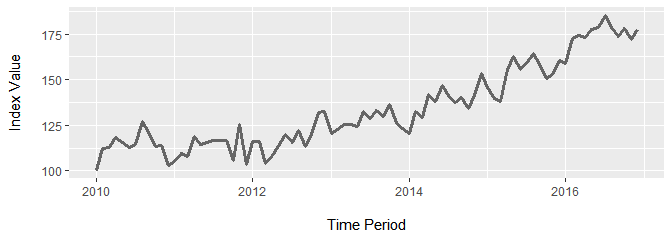The hedIndex() wrapper function will perform all of the above hedonic modeling tasks, and then condense the results into an unified object of class hpi. There are three ‘levels of entry’ with the wrapper function. 1. You can give it a raw data.frame with your transactions and it will A) Add the periods; B) Create a hedonic sales object; and 3) Model the data and create the index. Under this approach trans_df is passed a raw data.frame along with the following arguments, some of which are optional (will revert to default if not given) and some of which are required (mostly field names and the model specification): • periodicity (optional: default = ‘annual’) • min_date (optional: default = minimum of data) • max_date (optional: default = maximum of data) • adj_type (opt: default = ‘move’) • date (required) • price (required) • trans_id (required) • prop_id (required) • dep_var (required, unless hed_spec supplied) • ind_var (required, unless hed_spec supplied) • hed_spec (required, unless dep_var and ind_var supplied) • estimator (optional: default = ‘base’) • log_dep (optional: default = TRUE) • trim_model (optional: default = FALSE) • max_period (optional: default = maximum of data) • smooth (optional: default = FALSE) • smooth_order (optional, default = 3)  hed_1 <- hedIndex(trans_df = ex_sales, periodicity = 'monthly', min_date = '2010-06-01', max_date = '2015-11-30', adj_type = 'clip', date = 'sale_date', price = 'sale_price', trans_id = 'sale_id', prop_id = 'pinx', estimator = 'robust', log_dep = TRUE, trim_model = TRUE, max_period = 48, dep_var = 'price', ind_var = c('tot_sf', 'beds', 'baths'), smooth = FALSE) ## Supplied "min_date" date is greater than minimum of transactions. Clipping transactions. ## Supplied "max_date" is less than maximum of transactions. Clipping transactions. 1. You can provide an hpidata object (one that has been through the dateToPeriod() function). In this case, the following arguments are valid: • date (required) • price (required) • trans_id (required) • prop_id (required) • dep_var (required, unless hed_spec supplied) • ind_var (required, unless hed_spec supplied) • hed_spec (required, unless dep_var and ind_var supplied) • estimator (optional: default = ‘base’) • log_dep (optional: default = TRUE) • trim_model (optional: default = FALSE) • max_period (optional: default = maximum of data) • smooth (optional: default = FALSE) • smooth_order (optional, default = 3)  sales_hdf <- dateToPeriod(trans_df = ex_sales, date = 'sale_date', periodicity = 'monthly', min_date = '2010-02-01', max_date = '2015-11-30', adj_type = 'move') ## Supplied "min_date" is greater than minimum of transactions. Adjusting. ## Supplied "max_date" is less than maximum of transactions. Adjusting.  hed_2 <- hedIndex(trans_df = sales_hdf, date = 'sale_date', price = 'sale_price', trans_id = 'sale_id', prop_id = 'pinx', estimator = 'base', log_dep = FALSE, trim_model = FALSE, max_period = 56, dep_var = 'price', ind_var = c('tot_sf', 'beds', 'baths'), smooth = TRUE) 1. Finally, you can provide it an heddata object (one that has been through the hedCreateTrans() function). Only the following arguments can be given in this case. • dep_var (required, unless hed_spec supplied) • ind_var (required, unless hed_spec supplied) • hed_spec (required, unless dep_var and ind_var supplied) • estimator (optional: default = ‘base’) • log_dep (optional: default = TRUE) • trim_model (optional: default = FALSE) • max_period (optional: default = maximum of data) • smooth (optional: default = FALSE) • smooth_order (optional, default = 3)  sales_hhdf <- hedCreateTrans(trans_df = sales_hdf, prop_id = 'pinx', trans_id = 'sale_id', price = 'sale_price')  hed_3 <- hedIndex(trans_df = sales_hhdf, estimator = 'weighted', log_dep = TRUE, trim_model = FALSE, max_period = 80, dep_var = 'price', ind_var = c('tot_sf', 'beds', 'baths'), weights = runif(nrow(sales_hhdf), 0, 1), smooth = TRUE, smooth_order = 5) Again, we can plot with a simple plot() call.  plot(hed_3, smooth=TRUE)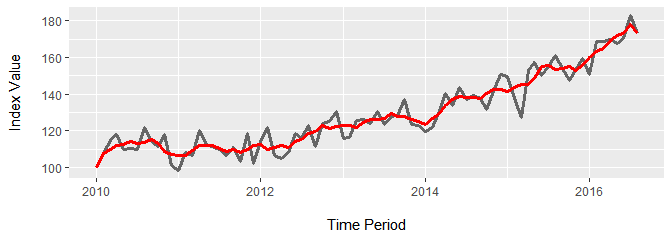### Analyzing an index Before we start analyzing our indexes, we’ll create two example hpi objects, one using a repeat sales model and one with a hedonic price model.  rt_hpi <- rtIndex(trans_df = sales_rtdf, estimator = 'robust', log_dep = TRUE, trim_model = FALSE, max_period = 84, smooth = TRUE) hed_hpi <- hedIndex(trans_df = sales_hhdf, estimator = 'weighted', log_dep = TRUE, trim_model = FALSE, max_period = 84, dep_var = 'price', ind_var = c('tot_sf', 'beds', 'baths'), weights = runif(nrow(sales_hhdf), 0, 1), smooth = TRUE) #### Volatility One thing we can check for an index is its volatility. We can get the a rolling window volatility (standard deviation) of the index with the calcIndexVolatility() function. The window over which to calculate the volatility can be specified. Both volatility metrics at each window calculation (\$roll) as well as the summarized average volality(\$mean) and median (\$median) are returned by the function.

  index_vol <- calcVolatility(index = hed_hpi$index$value,
window = 3)
names(index_vol)
##  "roll"   "mean"   "median"

Plotting an indexvolatility object shows the rolling volatility, the mean (dashed line) and the median (dotted) volatility on the top panel.

  plot(index_vol)
## Warning: Removed 3 rows containing missing values (geom_path).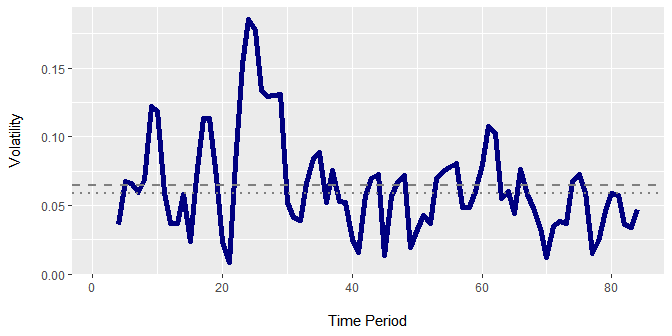A number of differently classed objects can be passed to the index argument in the calcVolatility() function. These include the extracted ts object from an hpiindex object (as done above), the raw hpiindex object itself (from modelToIndex()), or an hpi object from one of the wrappers (rtIndex() or hedIndex())

 # hpinindex object
index_vol <- calcVolatility(index = hed_hpi$index, window = 3) # hpi object index_vol <- calcVolatility(index = hed_hpi, window = 3) If you want to calculate the volatility of the smoothed index, you need to pass the smoothed index directly or change the smooth argument to TRUE (default is false) when passing an hpiindex or hpi object to calcVolatility(). Note, you must make sure that a ‘smoothed’ index exists in the hpi or hpindex object first.  # Direct passing sindex_vol <- calcVolatility(index = hed_hpi$index$smooth, window = 3) # While passing 'hpi' or 'hpiindex' sindex_vol <- calcVolatility(index = hed_hpi$index,
window = 3,
smooth = TRUE)

The calcVolatility() function can also place the volatility metrics directly into the hpiindex object by setting in_place = TRUE. If passed an hpiindex object, the results will be stored in the hpiindex object which is returned. If passed an hpi object, the results will be stored in the hpiindex object within the hpi object which is returned.

   # Add it to the 'hpiindex' object
hed_hpi$index <- calcVolatility(index = hed_hpi$index,
window = 3,
in_place = TRUE)

# Add it to the full 'hpi' object (to the hpiindex object)
hed_hpi <- calcVolatility(index = hed_hpi,
window = 3,
in_place = TRUE)

A volatility analysis of a smoothed index will place it under the $volatility_smooth name.  hed_hpi <- calcVolatility(index = hed_hpi, window = 3, in_place = TRUE, smooth = TRUE) names(hed_hpi$index)
##  "name"              "numeric"           "period"
##  "value"             "imputed"           "smooth"
##  "volatility"        "volatility_smooth"

If you want to add it to the hpiindex object under a different name – say if you are comparing volatilities of different windows – you can use the in_place_name argument to give it a different name than the defaults (‘volatility’ and ‘volatility_smoothed’)

   hed_index <- calcVolatility(index = hed_hpi$index, window = 3, in_place = TRUE, in_place_name = 'vol_3') names(hed_index) ##  "name" "numeric" "period" ##  "value" "imputed" "smooth" ##  "volatility" "volatility_smooth" "vol_3" A note on renaming and in place addition. All of the analytical objects that are added – volatility and other below – can be renamed to allow for multiple analyses with different parameters. Smoothed indexes, however, cannot be renamed and must be stored under the name ‘smooth’ because all of the analytical process depend on identifying this named slot. #### Fitting Accuracy (Index) Next, we can test the accuracy of an index. In this case, we will define accuracy as the ability to properly estimate the second transaction price of a repeat transaction pair (a property that sells twice).2 To start, we’ll do this in-sample, ignoring any issues of overfitting. The calcAccuracy() function requires a full hpi object as its main argument. test_type defines the manner of testing accuracy; at the moment the only working options is “rt” which uses repeat transactions to judge the accuracy of an index. (Additional types to come). test_method sets the manner in which the test is employed, “insample” or “kfold”. If the hpi_obj does not match the test_type (in all cases “rt” for now), then you must supply an rtdata object to in the pred_df argument. The calcAccuracy() function returns a data.frame comparing the actual (repeat) transaction price to the one predicted by the index. A "test_method" attribute indicates the type of accuracy test performed.  rt_is_accr <- calcAccuracy(hpi_obj = rt_hpi, test_type = 'rt', test_method = 'insample') attr(rt_is_accr, 'test_method') ##  "insample" The smoothed index (if present) can be analyzed by setting smooth = TRUE  rts_is_accr <- calcAccuracy(hpi_obj = rt_hpi, test_type = 'rt', test_method = 'insample', smooth = TRUE) As with the other analytical functions, the results of the accuracy calculations can be added to the hpi_obj by returning them in place (in_place == TRUE). A name (“default = ‘accuracy’”") may also be given.  # Returns an accuracy object in place hed_hpi <- calcAccuracy(hpi_obj = hed_hpi, test_type = 'rt', test_method = 'insample', pred_df = sales_rtdf, in_place = TRUE, in_place_name = 'is_accuracy') names(hed_hpi) ##  "data" "model" "index" # Returns a smooth accuracy object in place hed_hpi <- calcAccuracy(hpi_obj = hed_hpi, test_type = 'rt', test_method = 'insample', smooth = TRUE, pred_df = rt_hpi$data,
in_place = TRUE,
in_place_name = 'smooth_is_accuracy')

To create a better estimate of predictive ability we can employ a K-fold, out of sample test of accuracy. In a K-fold test, some percentage of the observations are withheld, the index re-estimated and then the newly estimated index is judged by its ability to predict the repeat transaction price in the withheld (out-of) sample.

Two additional arguments are availabile here: 1) k - the number of folds to make (default = 10); and 2) seed a value to the control the random sampling (default = 1).

  rt_kf_accr <- calcAccuracy(hpi_obj = rt_hpi,
test_type = 'rt',
test_method = 'kfold',
k = 10,
seed = 123)

We can do this with smoothed indexes.

  rt_kf_accr_s <- calcAccuracy(hpi_obj = rt_hpi,
test_type = 'rt',
test_method = 'kfold',
k = 10,
seed = 123,
smooth = TRUE)

We can also return these in place.

    hed_hpi <- calcAccuracy(hpi_obj = hed_hpi,
test_type = 'rt',
test_method = 'kfold',
k = 10,
seed = 1,
pred_df = rt_hpi$data, in_place = TRUE, in_place_name = 'kf_accuracy') A plotting method (plot.hpiaccuracy()) shows a number of diagnostics about the accuracy analysis. Because the plotting object here contains multiple plot objects, if you wish to save this plot to a named object, you need to add return_plot = TRUE to the argument list.  plot(hed_hpi$index$kf_accuracy)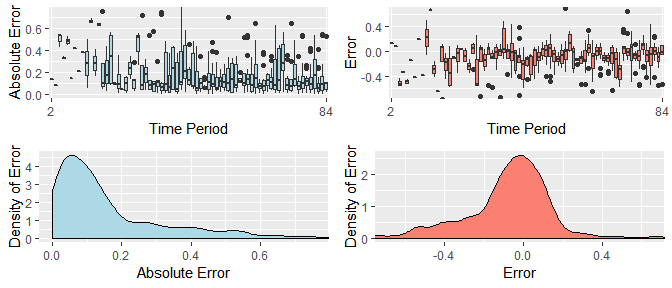### Index Series Two other aspects or metrics of house price indexes are revision and forecast accuracy. Forecast accuracy refers to how well an index forecast captures future market movements. Revision refers to how much original index values change over time as new data is accumulated (new transactions occur). Both concepts are described more fully below. To calculate either of these metrics requires a series of indexes. A series of indexes is a collection of progressively longer indexes that are estimated over time, or estimated sequentially such that the knowledge of the future is withheld from the model. For example, imagine we have, as the example below does, 84 months (periods) of data. Since we are using a statistical modeling approach, let’s assume that we need at least 12 period of data (training period) to fit a model. We begin by creating an index for periods 1 through 12, assuming that we only have data from periods 1 through 12. Then we do 1 through 13, assuming we only have data from periods 1 through 13; on up to period 84. This represents an index series. The indexes in the series are identified by the number of the last period that they are estimating, index 12, index 13, etc. The createSeries() function will create these series. The hpi_obj argument must be a full hpi object (created with one of the index wrappers). train_period sets how many periods to use for initial training (length of the shortest period). The max_period argument (default is the entire length of existing index) will control the end period to estimate a series up to (defaults to the original length of the index in the hpi object.). The createSeries() function will return an object of class serieshpi (data plus a series of hpi objects).  rt_series <- createSeries(hpi_obj = rt_hpi, train_period = 24, max_period = 30) A series can be plotted with the plot.serieshpi() method.  plot(rt_series)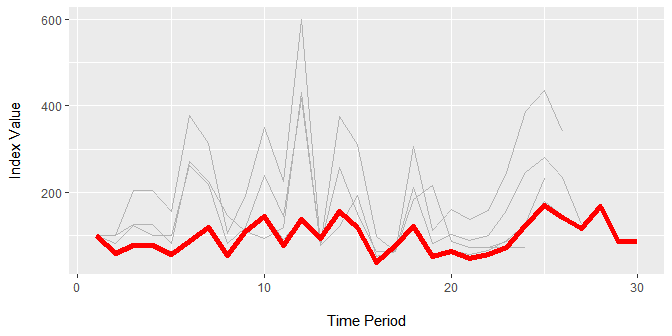#### Series Analysis A wrapper function, smoothSeries() will smooth all hpiindex objects in a series. The smoothing will occur “in place” and a series object will be returned by the function.  rt_series <- smoothSeries(series_obj = rt_series, order = 5) A wrapper function, calcSeriesVolatility() will calculate volatility for all hpiindex objects in a series. The calculations will occur “in place” and a series object will be returned by the function. Can be done on smoothed indexes as well (smooth = TRUE)  rt_series <- calcSeriesVolatility(series_obj = rt_series, window = 3, smooth = TRUE)  A wrapper function, calcSeriesAccuracy() will calculate accuracy for all hpiindex objects in a series and return to an object of class seriesaccuracy. Smoothed indexes can be evaluated as well (smooth = TRUE)  rt_sacc <- calcSeriesAccuracy(series_obj = rt_series, test_method = 'insample', test_type = 'rt') class(rt_sacc) ##  "seriesaccuracy" "hpiaccuracy" "data.frame" These calculations can occur “in place” and a series object will be returned by the function. Note that running this function on the “kfold” method may be computationally intense depending on your data size and index length. Unlike index accuracy calculations which are saved in the hpiindex object, series accuracy calculations are saved in the serieshpi object when done “in place”.  rt_series <- calcSeriesAccuracy(series_obj = rt_series, test_method = 'kfold', test_type = 'rt', smooth = TRUE, in_place=TRUE) One thing to note about series accuracy is that the training data will be evaluated separated for each index in the series, meaning that there will be many prediction errors for each observation. By setting summarize = TRUE, the mean for each observation will be calculated.  series_acc_summ <- calcSeriesAccuracy(series_obj = rt_series, test_method = 'insample', test_type = 'rt', summarize = TRUE) nrow(series_acc_summ) ##  35 nrow(rt_series$accuracy)
##  189

#### Forecast Accuracy

A final way to estimate accuracy is with a forecast test. In a forecast test, we use a series of indexes and continue to predict one period ahead and measure how well the predicted index does against repeated transactions in that period. In highly volatile or cyclical markets this is the most representative method of the answering the question: “how well does this index represent the near future?”

As above, it can be used on the raw index values or the smoothed ones (smooth = TRUE). The forecast accuracy tests are saved in the serieshpi object.

  rt_series <- calcSeriesAccuracy(series_obj = rt_series,
test_method = 'forecast',
test_type = 'rt',
smooth = TRUE,
in_place = TRUE)

We can plot the seriesaccuracy objects, which look nearly identical to hpiaccuracy objects.

  plot(rt_series\$accuracy_smooth)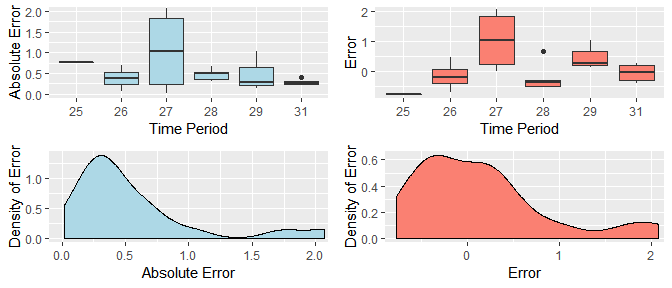#### Revision

As can be the case with house price indexes, the estimated index values move considerably as new data (transactions) are accumulated over time. We can measure the amount of this change, or ‘revision’ over time as a useful metric or property of the series or of the final index.

Within the literature on house price indexes there is no standard or agreed upon metric to summarize revision. Here we do it two ways. First, at the period level where we calculate the average revision (change from period to period) for each period in the series of indexes. Obviously, the earlier period have a larger sample size (though they do have more time to be influenced by future transactions) than the latter periods are and, as such, are likely to be less volatile.

The second calculation simply averages all revisions across all periods to provide a single value of the average and median revision for the index series.


# Return to a revision object
rt_rev <- calcRevision(series_obj = rt_series)

# Return in place (with smooth)
rt_series <- calcRevision(series_obj = rt_series,
in_place = TRUE,
smooth = TRUE)
names(rt_series)
##  "data"            "hpis"            "accuracy_smooth" "revision_smooth"

We can plot the revisions with the plot.hpirevision() method to show the average revision by time period. The measure argument (default = “median”) determines whether median or mean revisions will be shown.

  plot(rt_rev, measure='median')`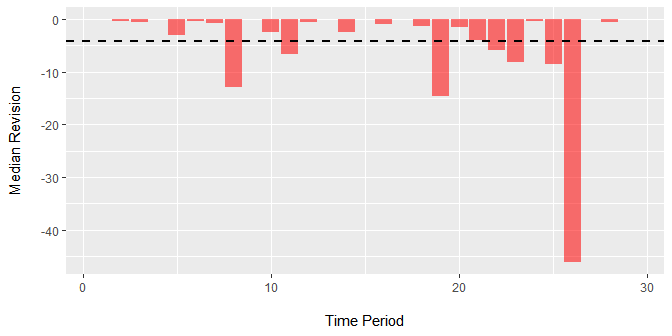More information on the class structures of the object created above can be found in the “Classes in hpiR” vignette in this package.

1. A small amount of data cleaning has gone into the creating the data, for more information on this process please contact the author.

2. Note that a second method using hedonic pricing models is being developed.